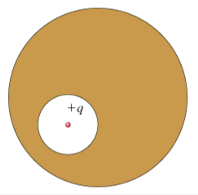# Problem: A spherical cavity is hollowed out of the interior of a neutral conducting sphere. At the center of the cavity is a point charge, of positive charge q.What is the electric field E_ext outside the conductor?(a) Zero(b) The same as the field produced by a point charge q located at the center of the sphere.(c) The same as the field produced by a point charge located at the position of the charge in the cavity.

###### FREE Expert Solution

Gauss' law:

$\overline{){{\mathbf{\varphi }}}_{{\mathbf{E}}}{\mathbf{=}}{\mathbf{\oint }}{\mathbf{E}}{\mathbf{·}}{\mathbf{d}}{\mathbf{A}}{\mathbf{=}}\frac{{\mathbf{q}}_{\mathbf{enc}}}{{\mathbf{\epsilon }}_{\mathbf{0}}}}$

The flux is the same because the charge enclosed has not changed.###### Problem Details

A spherical cavity is hollowed out of the interior of a neutral conducting sphere. At the center of the cavity is a point charge, of positive charge q.What is the electric field E_ext outside the conductor?

(a) Zero

(b) The same as the field produced by a point charge q located at the center of the sphere.

(c) The same as the field produced by a point charge located at the position of the charge in the cavity.